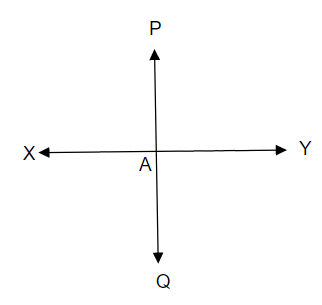# Let $\overline{\mathrm{PQ}}$ be the perpendicular to the line segment $\overline{\mathrm{XY}}$. Let $\overline{\mathrm{PQ}}$ and $\overline{\mathrm{XY}}$ intersect in the point $\mathrm{A}$. What is the measure of $\angle \mathrm{PAY}$ ?

Given:

$\overline{\mathrm{PQ}}$ is perpendicular to the line segment $\overline{\mathrm{XY}}$.

$\overline{\mathrm{PQ}}$ and $\overline{\mathrm{XY}}$ intersect in the point $\mathrm{A}$.

To do:

We have to find the measure of $\angle \mathrm{PAY}$.
Solution:From the figure,

The measure of $\angle \mathrm{PAY}$ is $90^o$.